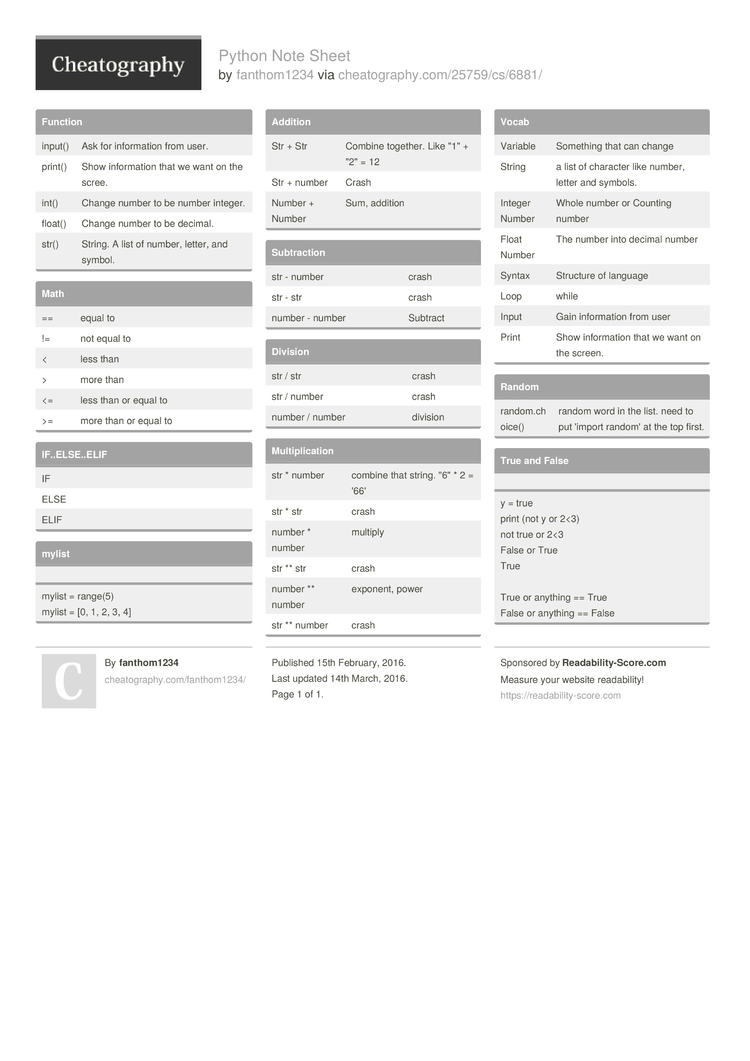# Python Note Sheet by fanthom1234

### Function

 input() Ask for inform­ation from user. print() Show inform­ation that we want on the scree. int() Change number to be number integer. float() Change number to be decimal. str() String. A list of number, letter, and symbol.

### Math

 == equal to != not equal to < less than > more than <= less than or equal to >= more than or equal to

### IF..EL­SE..ELIF

 IF ELSE ELIF

### mylist

mylist = range(5)
mylist = [0, 1, 2, 3, 4]

 Str + Str Combine together. Like "­1" + "­2" = 12 Str + number Crash Number + Number Sum, addition

### Subtra­ction

 str - number crash str - str crash number - number Subtract

### Division

 str / str crash str / number crash number / number division

### Multip­lic­ation

 str * number combine that string. "­6" * 2 = '66' str * str crash number * number multiply str ** str crash number ** number exponent, power str ** number crash

### Vocab

 Variable Something that can change String a list of character like number, letter and symbols. Integer Number Whole number or Counting number Float Number The number into decimal number Syntax Structure of language Loop while Input Gain inform­ation from user Print Show inform­ation that we want on the screen.

### Random

 random.ch­oice() random word in the list. need to put 'import random' at the top first.

### True and False

y = true
print (not y or 2<3)
not true or 2<3
False or True
True

True or anything == True
False or anything == False1 Page
//media.cheatography.com/storage/thumb/fanthom1234_python-note-sheet.750.jpg

PDF (recommended)